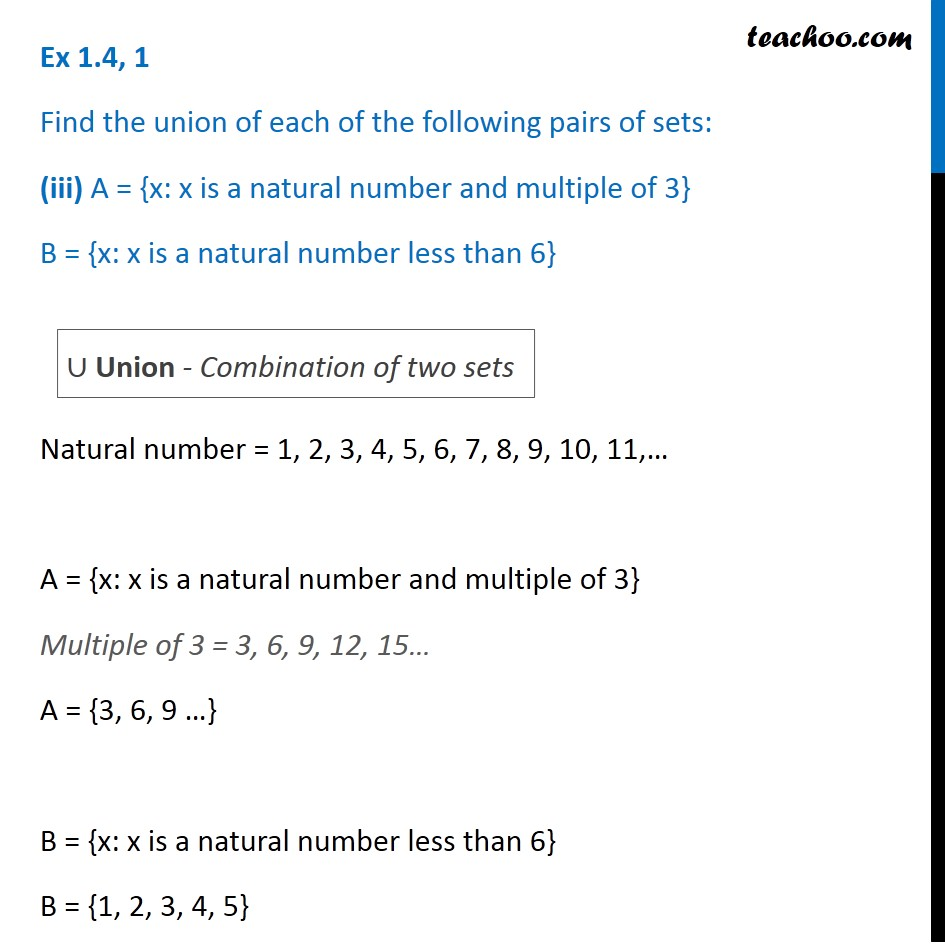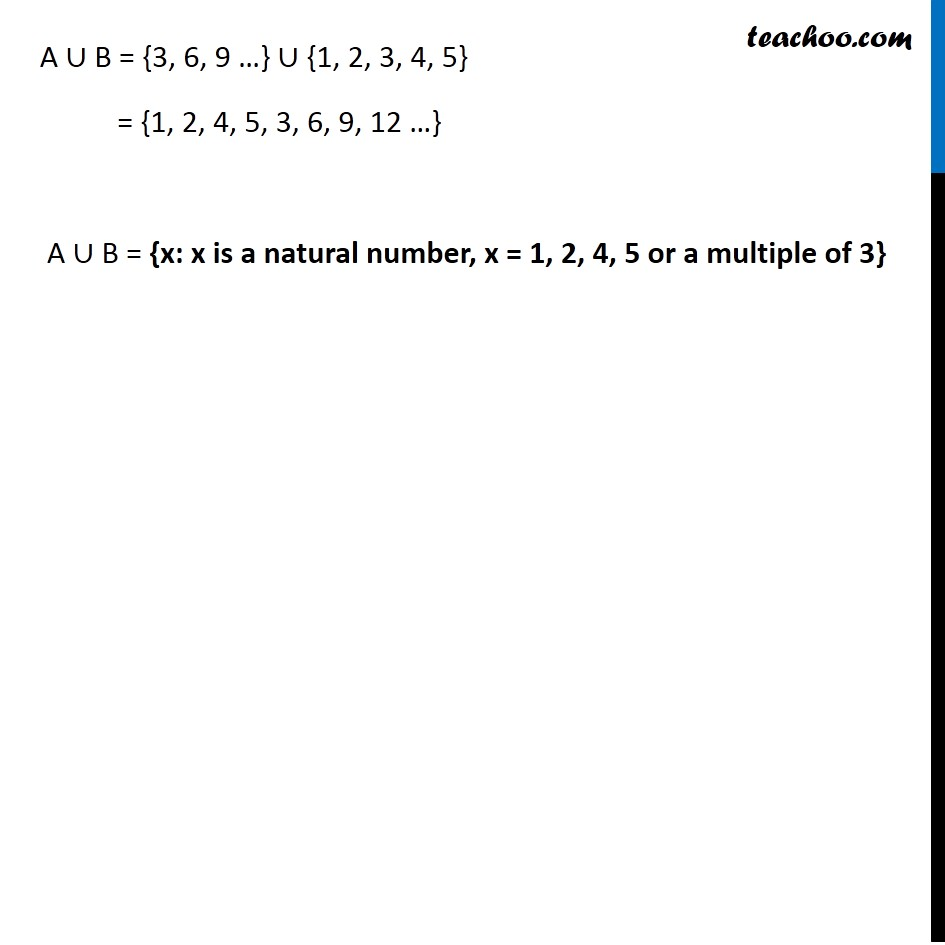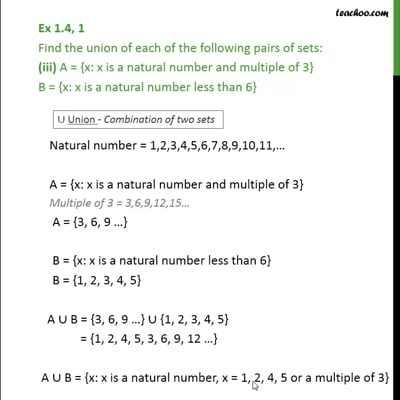Ex 1.4

Chapter 1 Class 11 Sets
Serial order wiseThis video is only available for Teachoo black users

Maths Crash Course - Live lectures + all videos + Real time Doubt solving!

### Transcript

Ex 1.4, 1 Find the union of each of the following pairs of sets: (iii) A = {x: x is a natural number and multiple of 3} B = {x: x is a natural number less than 6} Natural number = 1, 2, 3, 4, 5, 6, 7, 8, 9, 10, 11,… A = {x: x is a natural number and multiple of 3} Multiple of 3 = 3, 6, 9, 12, 15… A = {3, 6, 9 …} B = {x: x is a natural number less than 6} B = {1, 2, 3, 4, 5} ∪ Union - Combination of two sets A ∪ B = {3, 6, 9 …} ∪ {1, 2, 3, 4, 5} = {1, 2, 4, 5, 3, 6, 9, 12 …} A ∪ B = {x: x is a natural number, x = 1, 2, 4, 5 or a multiple of 3}